# AP Chemistry : Equilibrium Constant and Reaction Quotient

## Example Questions

← Previous 1

### Example Question #8 : Chemical Equilibrium

Which of the following can always be determined based on the equation itself?

The concentration of products

The equilibrium constant expression

The concentration of reactants

The rate law

The equilibrium constant expression

Explanation:

According to the law of mass action, the equilibrium constant expression can always be written given the equation of the reaction itself. The equilibrium-constant expression will be written as the products over the reactants, each raised to their respectively stoichiometric coefficient. The rate law and concentrations can only be determined if there is additional data given.

### Example Question #9 : Chemical Equilibrium

What is the difference between the reaction quotient and the equilibrium constant?

The equilibrium constant is valid only at equilibrium, and the reaction quotient can be calculated at any given moment in the reaction.

There is no difference between the two.

The reaction quotient is equivalent to the rate law.

The reaction quotient  is valid only at equilibrium, and the equilibrium constant can be calculated at any given moment in the reaction.

The equilibrium constant is valid only at equilibrium, and the reaction quotient can be calculated at any given moment in the reaction.

Explanation:

The correct answer gives the accurate definition of both the equilibrium constant and the reaction quotient.

### Example Question #1 : Equilibrium Constant And Reaction Quotient

The law of mass action gives an expression that is specific for a certain __________.

temperature

physical state

pressure

volume

temperature

Explanation:

Equilibrium expressions are specific for certain temperatures. Physical state, pressure, and volume do not factor into the expression.

### Example Question #2 : Equilibrium Constant And Reaction Quotient

Why do the concentrations of pure liquids and solids not appear in the equilibrium constant expression?

They are completely consumed before the reaction reaches equilibrium.

They are not present in the mixture at equilibrium.

Their concentrations change too quickly to be accounted for.

Their concentrations don't change over the course of the reaction.

Their concentrations don't change over the course of the reaction.

Explanation:

This is one of the properties of the law of mass action—the concentrations of pure solids and liquids don't change over the course of the reaction.

### Example Question #3 : Equilibrium Constant And Reaction Quotient

Which of the following is not a property of the law of mass action?

The larger the equilibrium constant (K), the farther to the right equilibrium lies.

A system shifts to reduce applied "stress."

The concentrations of pure solids and liquids don't appear in the equilbrium expression.

The equlibrium constant is characteristic of a particular reaction at a certain temperature.

A system shifts to reduce applied "stress."

Explanation:

All of the statements are properties of the law of mass action, except for the answer, which is a statement of Le Chatelier's Principle.

### Example Question #4 : Equilibrium Constant And Reaction Quotient

If the equilibrium constant lies farther to the right, this indicates that the reaction __________.

is less complete

does not have a bearing on the reaction

is more complete

includes a catalyst

is more complete

Explanation:

The equilibrium constant is given by the concentraton of products over the concentration of reactants. If it lies to the right, it means that it favors the forward reaction, and thus the reaction is "more complete" or closer to completion.

### Example Question #6 : Help With Equilibrium Constant

What is the equilbrium constant for a reaction written in reverse if the forward reaction has constant K?

4K

1/K

K/2

2K

1/K

Explanation:

This is one of the properties of the law of mass action. The equilbrium constant for a reaction written in reverse is 1/K.

### Example Question #7 : Help With Equilibrium Constant

Which of the following factors will change the equilibrium constant, Keq?

Change in solvent volume

Change in temperature

Adding a chemical that will cause side reactions

Change in temperature

Explanation:

The only factor that changes the equilibrium constant is temperature. Changes in concentration of reactants or products by any means (whether addition, taking away solvent, or adding a chemical that will cause side reactions) will remove the system from equilibrium, but will not change the equilibrium constant.

### Example Question #5 : Equilibrium Constant And Reaction Quotient

Consider the following reaction.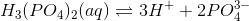What is the reaction quotient if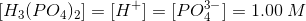?

There is not enough information to answer this question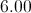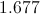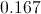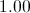Explanation: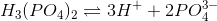The reaction quotient, or Q, of the above reaction is equal to the products over the reactants. Q is calculated in the same manner as Keq, but does not require that the reaction be at equilibrium.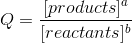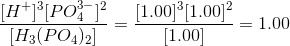### Example Question #6 : Equilibrium Constant And Reaction Quotient

Consider the following balanced reaction.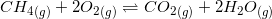Write the equilibrium constant expression for this reaction.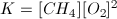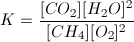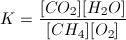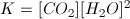Explanation:

When writing an equilibrium constant expression, remember that products are on the top, and reactants are on the bottom of the expression. The coefficients for the compounds in the balanced reaction become the exponents for the compounds seen in the expression.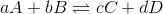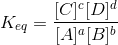← Previous 1